19 图像分割（上）：详解图像分割原理与图像分割模型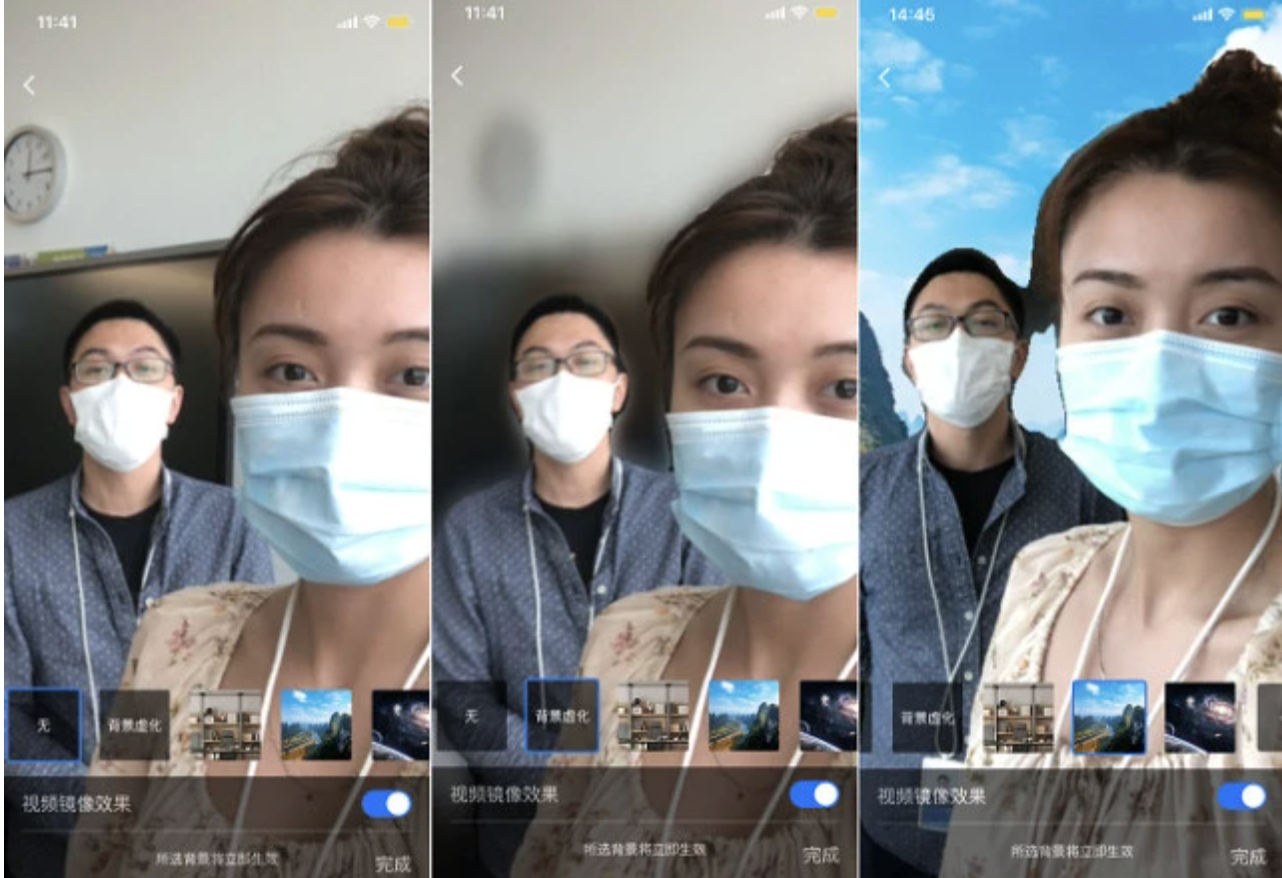## 图像分割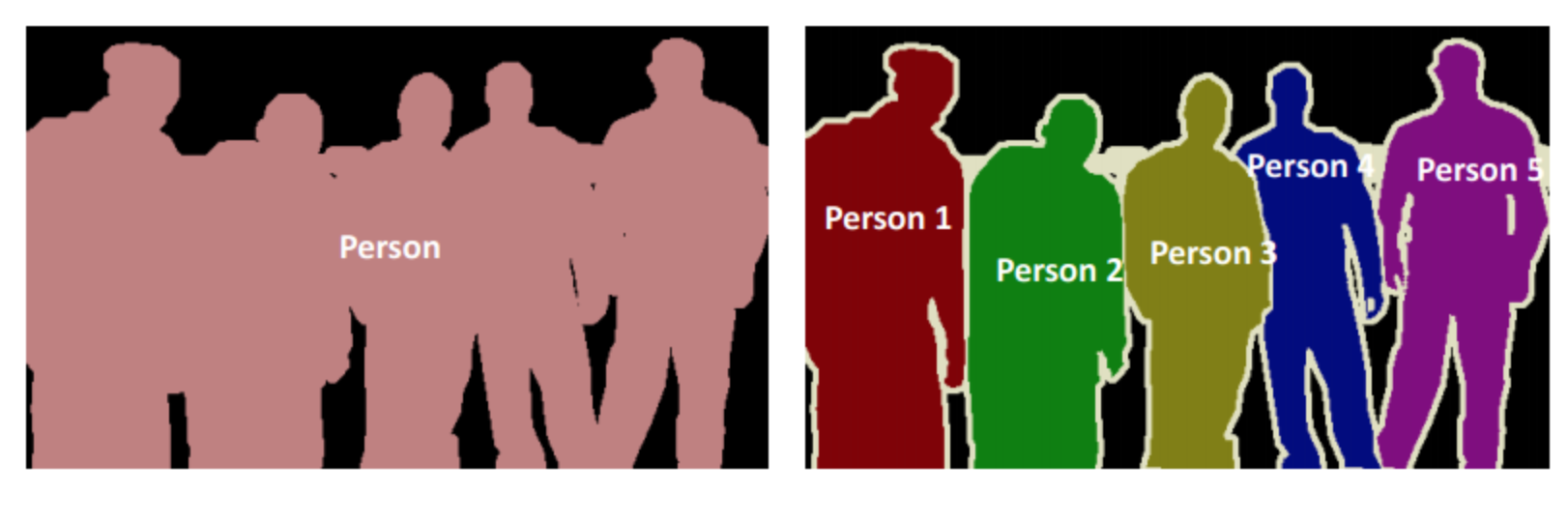## 语义分割原理

### 分类端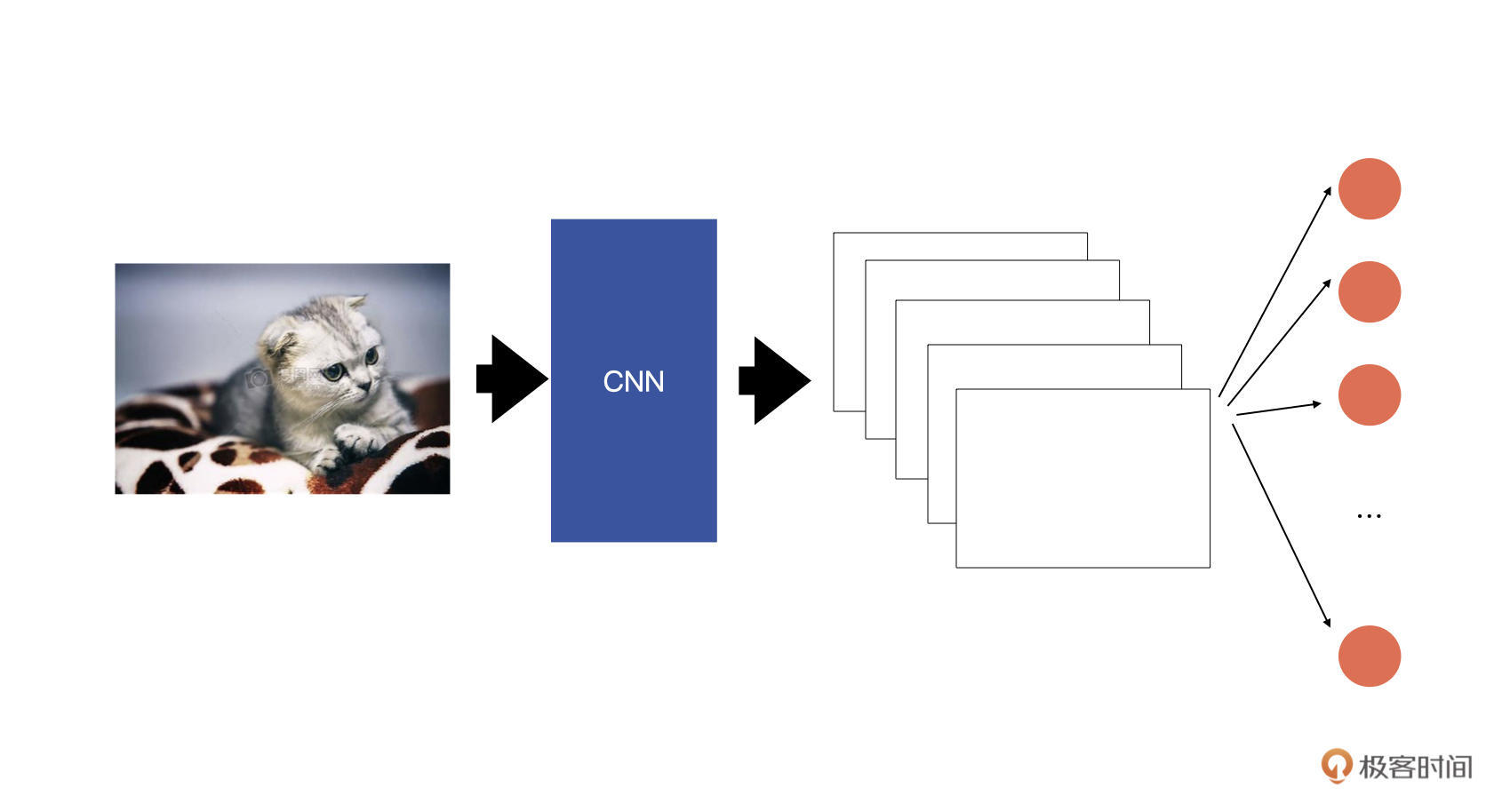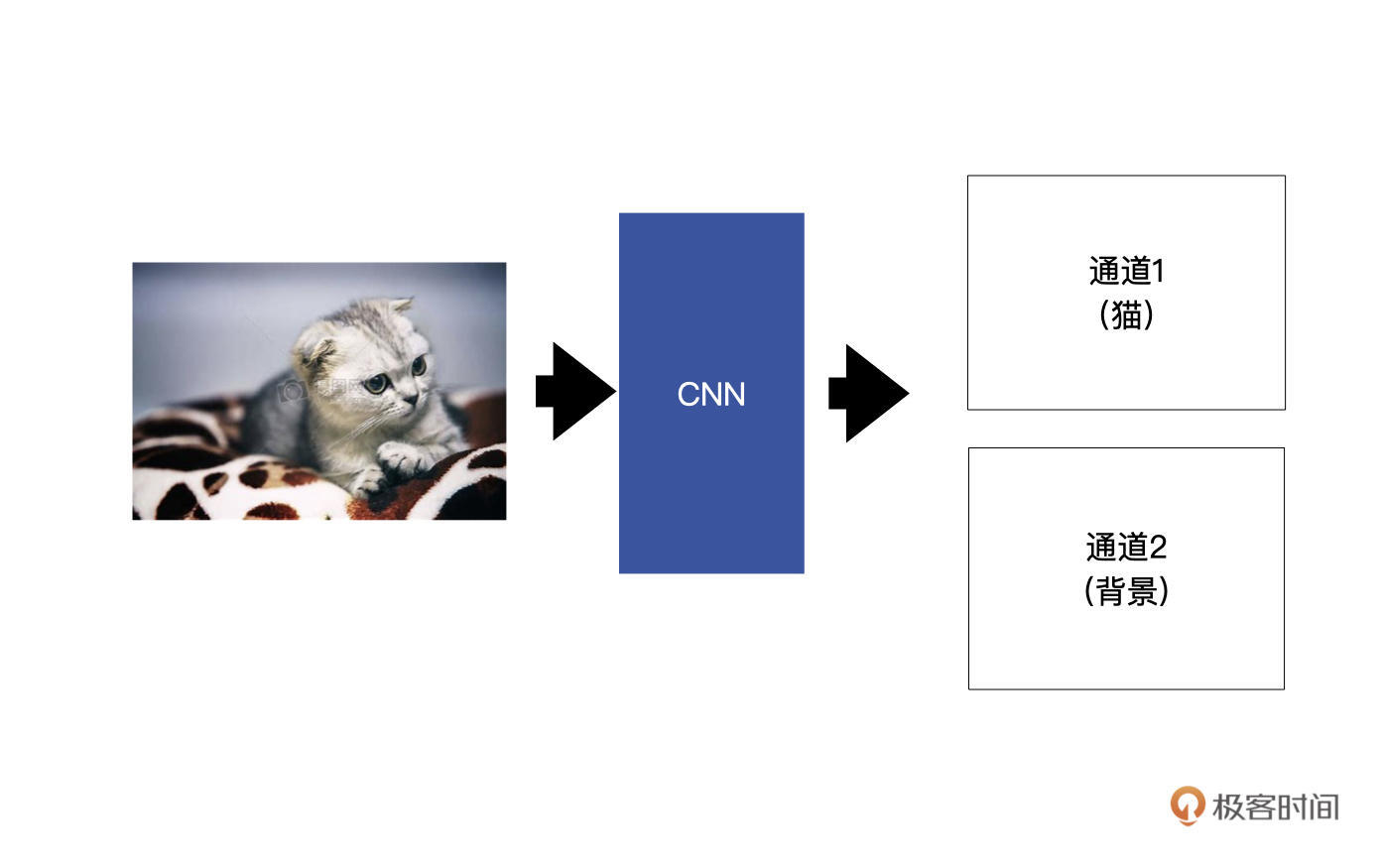### 网络结构

Decoder的作用就是对特征图尺寸进行还原的过程，将尺寸还原到一个比较大的尺寸。这个还原的操作对应的就是上采样。而在上采样中我们通常使用的是转置卷积。

#### 转置卷积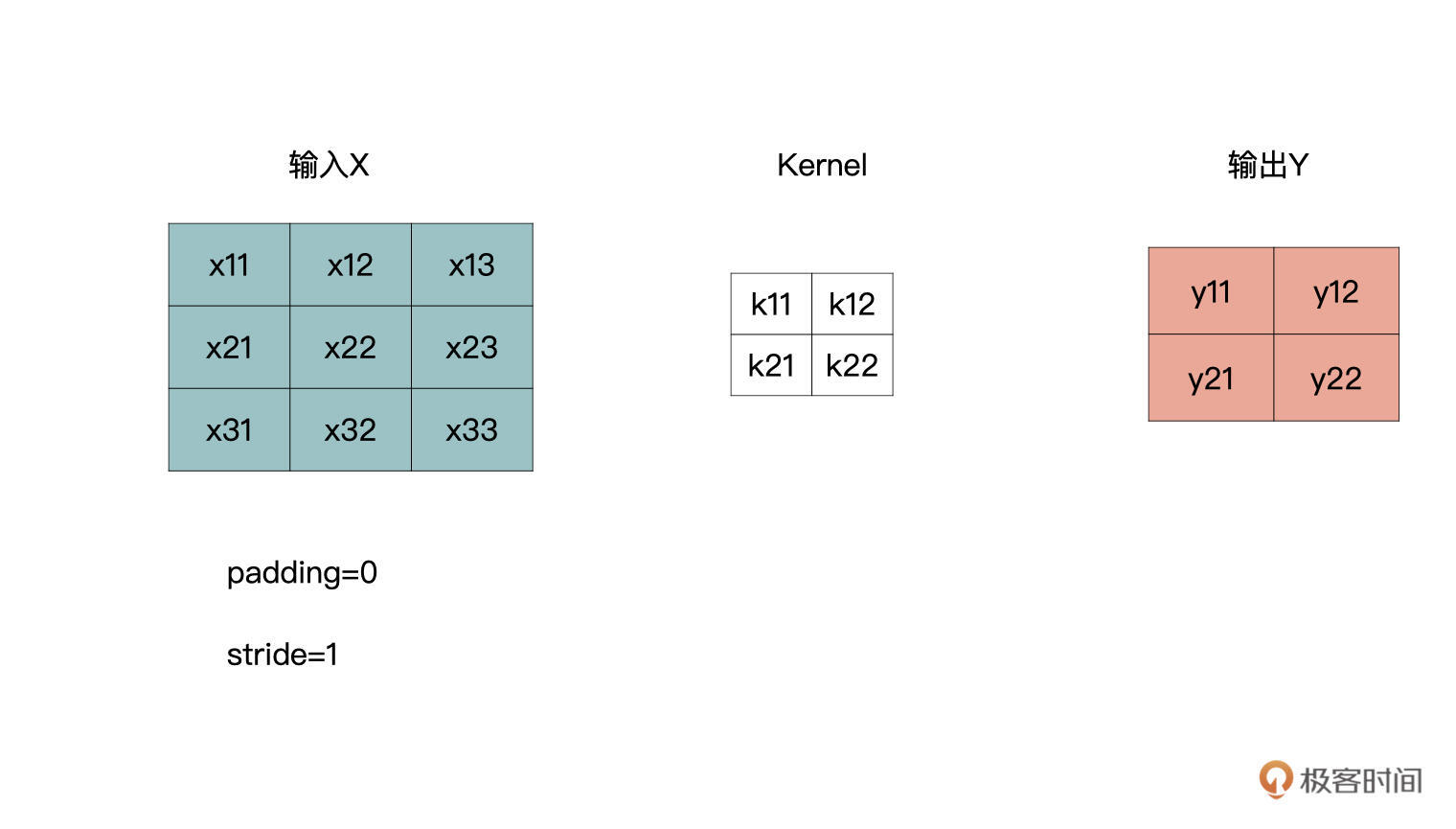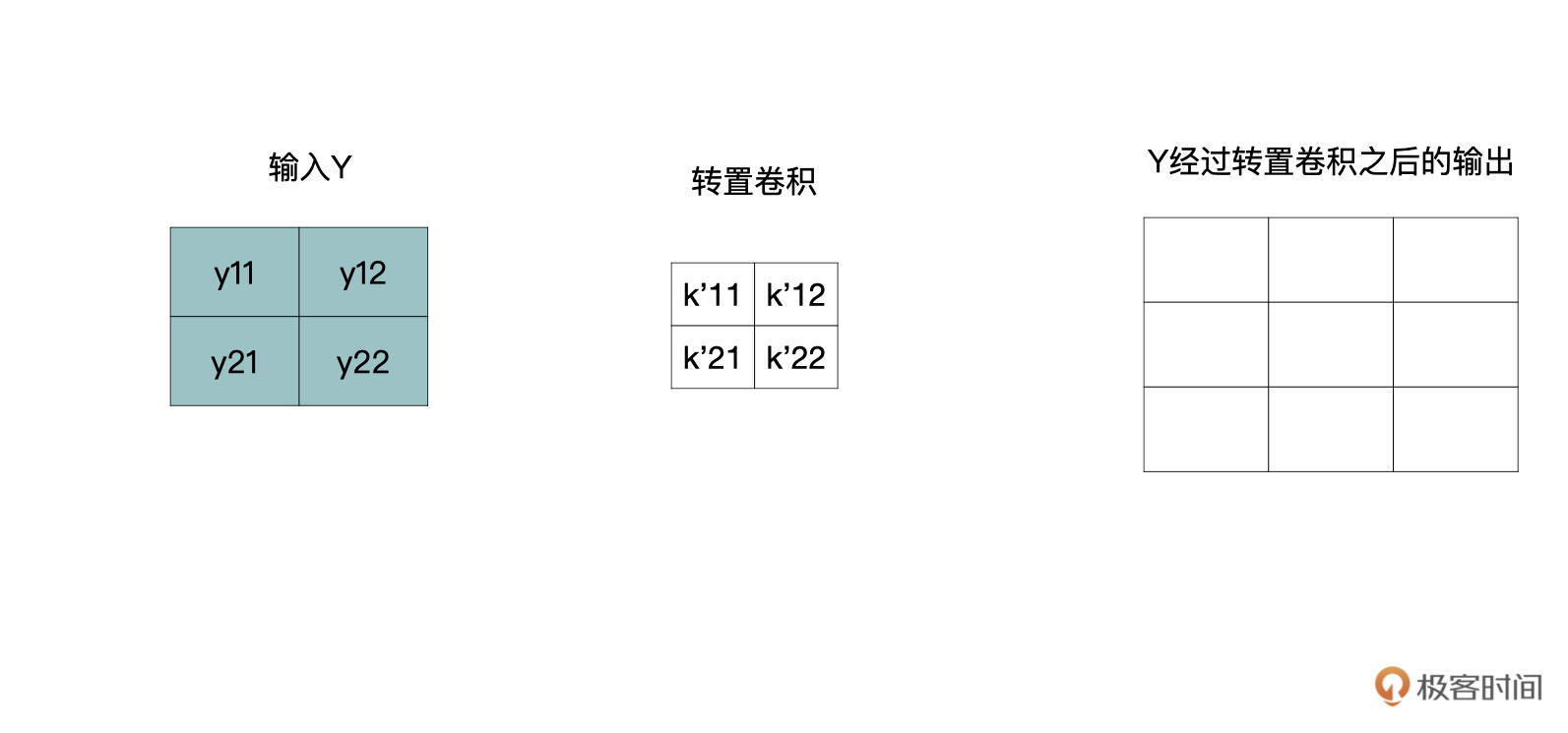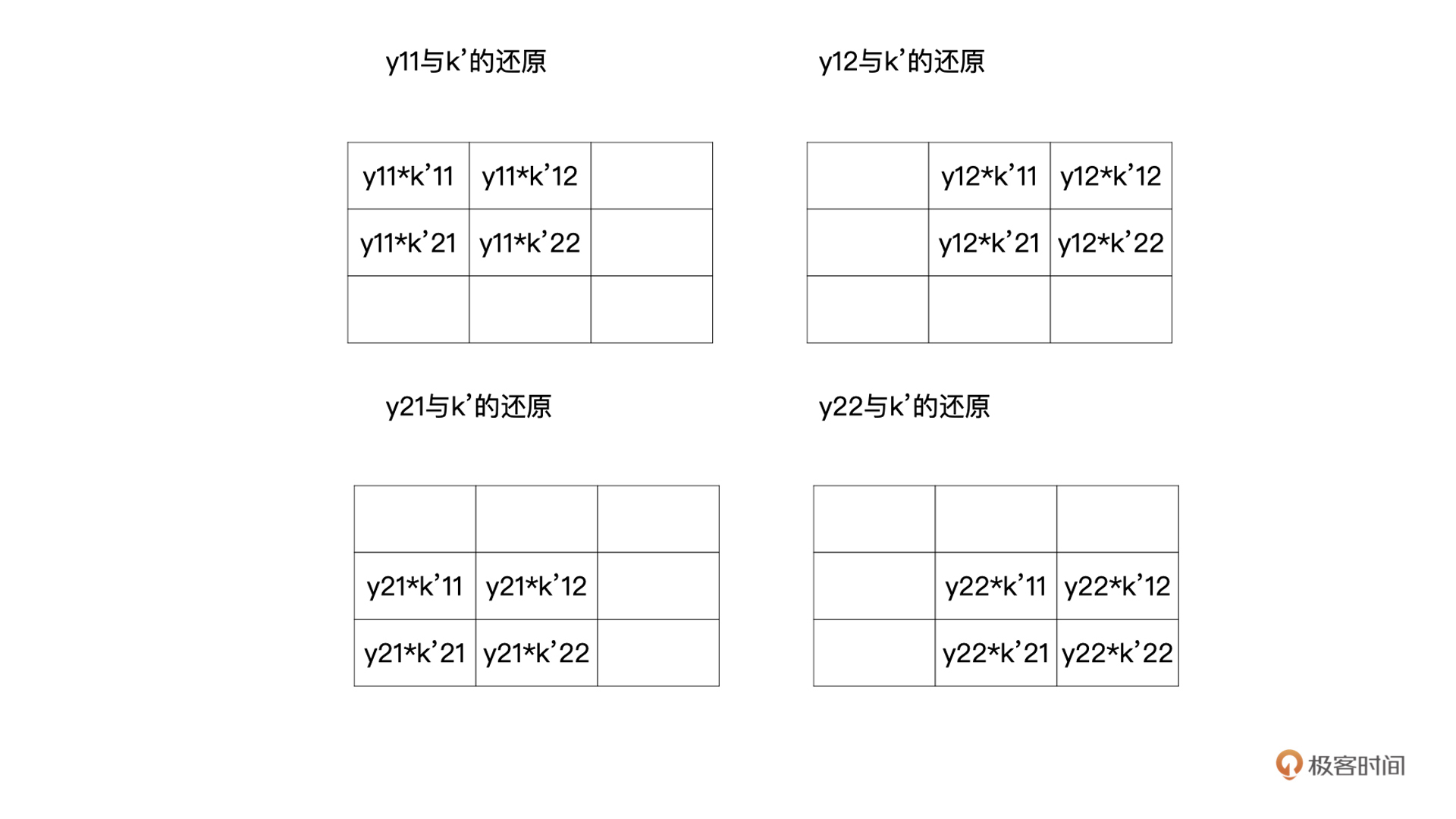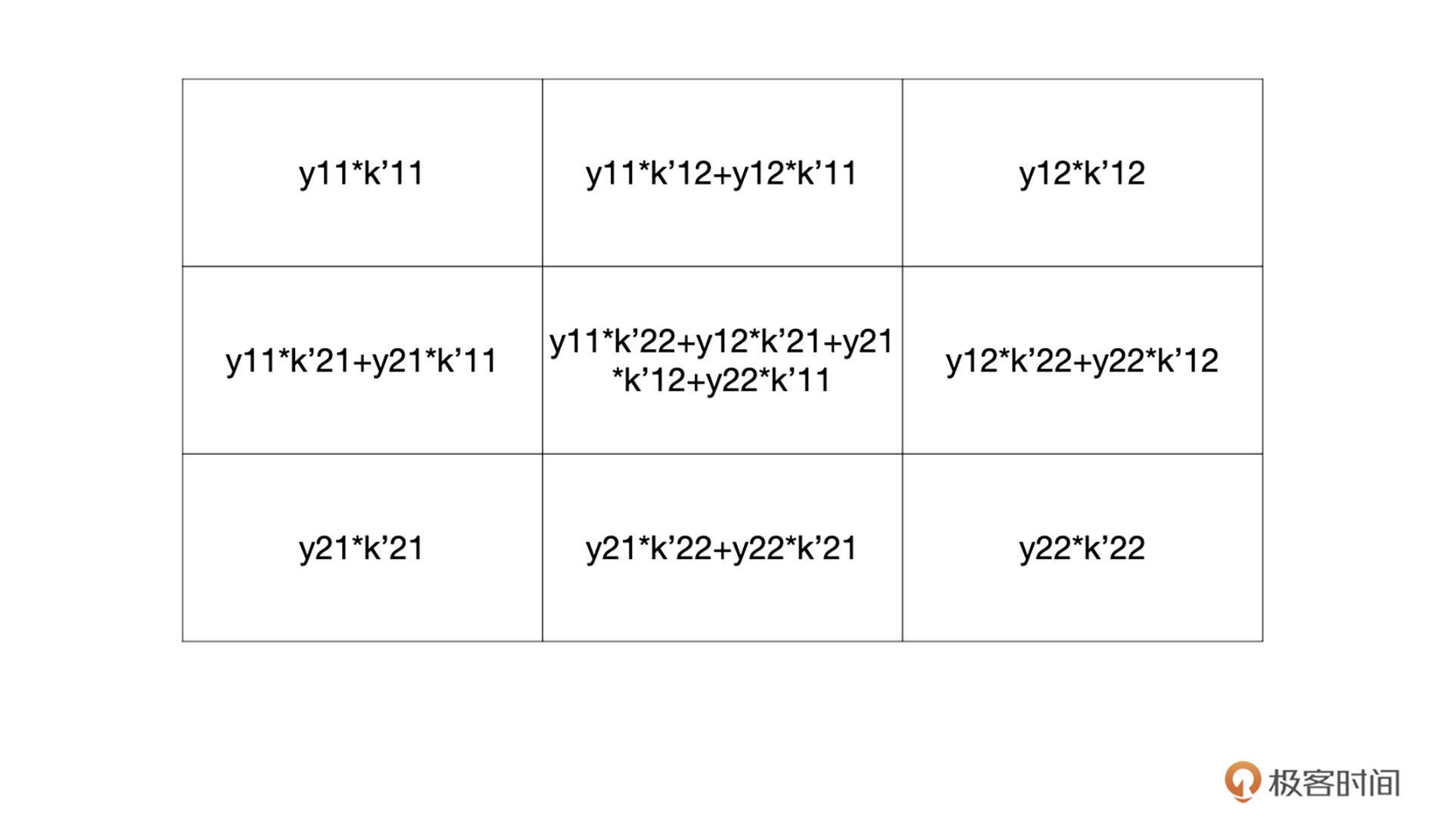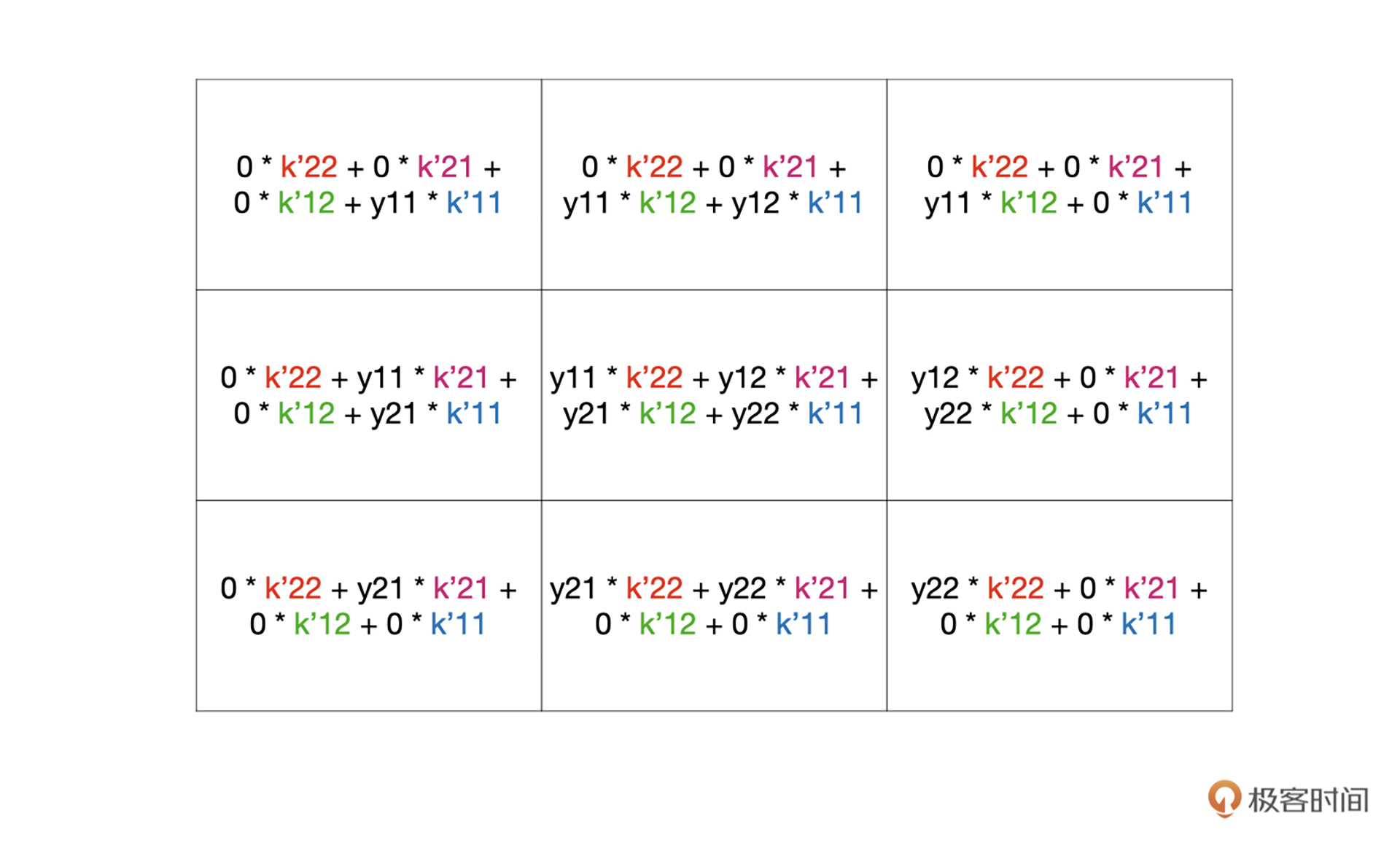class torch.nn.ConvTranspose2d(in_channels,
out_channels,
kernel_size,
stride=1,
groups=1,
bias=True,
dilation=1)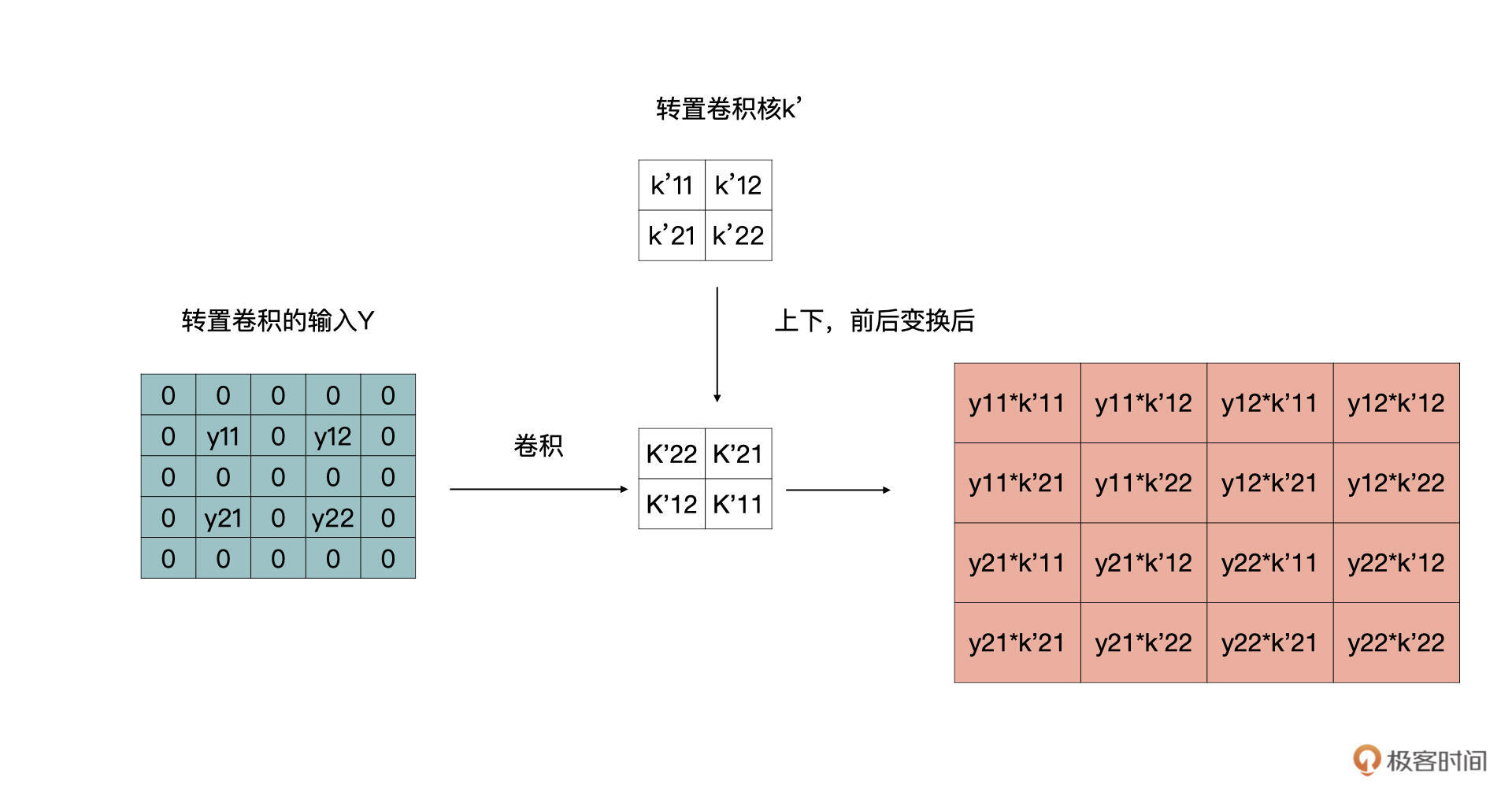$h\_{out} = (h\_{in} - 1) \* stride\[0$ - padding$0$ + kernel\\\_size$0$\]

$w\_{out} = (w\_{in} - 1) \* stride\[1$ - padding$1$ + kernel\\\_size$1$\]

import torch
import torch.nn as nn
import numpy as np
input_feat = torch.tensor([[[[1, 2], [3, 4]]]], dtype=torch.float32)
input_feat

tensor([[[[1., 2.],
[3., 4.]]]])


kernels = torch.tensor([[[[1, 0], [1, 1]]]], dtype=torch.float32)
kernels

tensor([[[[1., 0.],
[1., 1.]]]])



convTrans = nn.ConvTranspose2d(1, 1, kernel_size=2, stride=1, padding=0, bias = False)
convTrans.weight=nn.Parameter(kernels)


$input\\\_feat = \\begin{bmatrix}- 0 & 0 & 0 & 0 \\\\\\- 0 & 1 & 2 & 0 \\\\\\- 0 & 3 & 4 & 0 \\\\\\- 0 & 0 & 0 & 0\\\\\\- \\end{bmatrix}$

convTrans(input_feat)

tensor([[[[1., 2., 0.],
[4., 7., 2.],


### 损失函数

GT是Ground Truth的缩写，在图像分割中我们经常使用这个词。在图像分类中与之对应的就是数据的真实标签，在图像分割中则GT是每个像素的真实分类，如下面的例子所示。GT如下所示：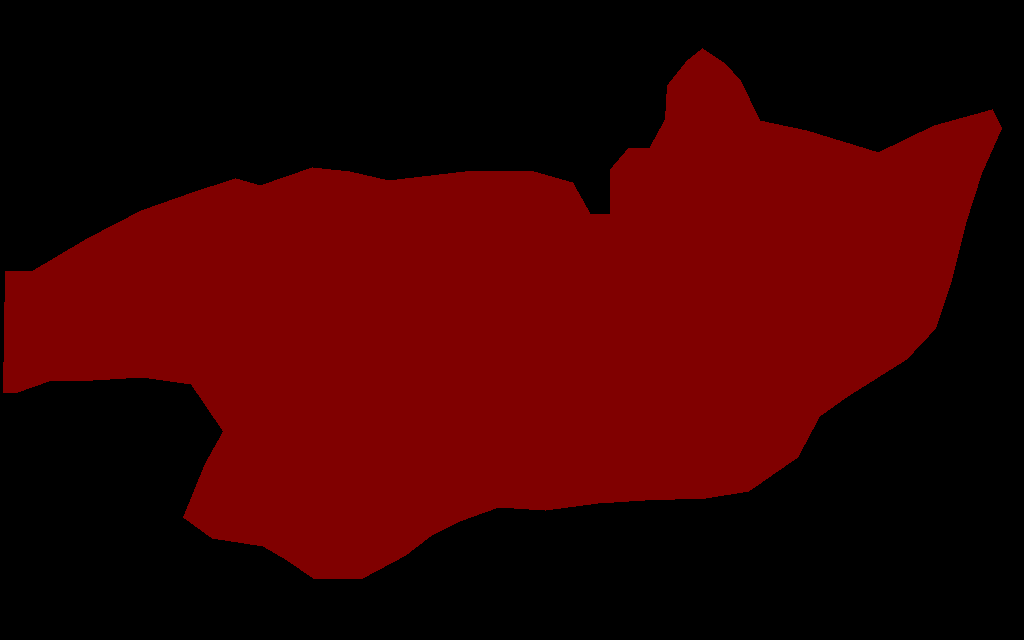#### 公开数据集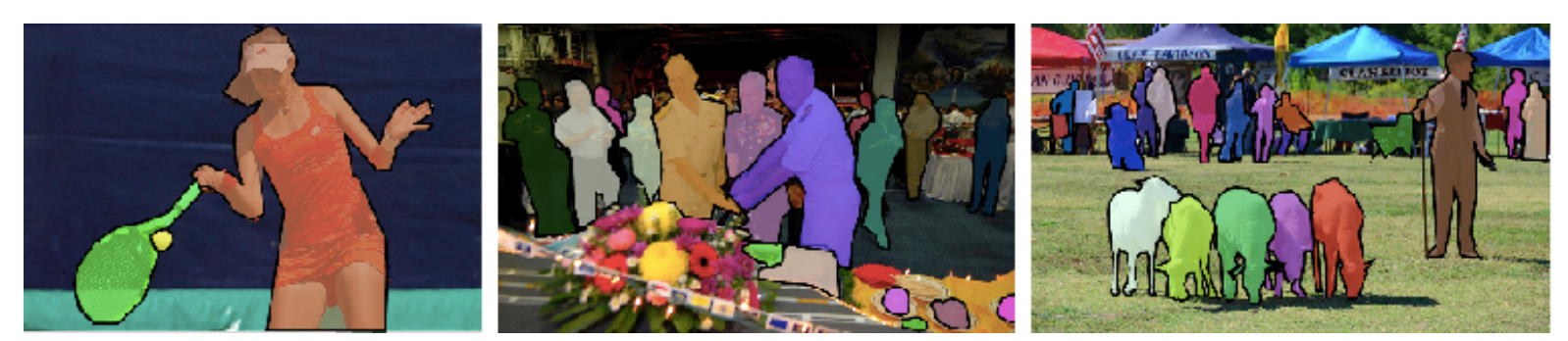## 小结

1.在分类端有所不同，在图像分类中，经过卷积的特征提取后，最后会以若干个神经元的形式作为输出，每个神经元代表着对一个类别的判断情况。而语义分割，则是会输出若干的特征图，每个特征图代表着对应类别判断。

2.在图像分类的网络中，特征图是不断减小的。但是在语义分割的网络中，特征图还会有decoder这一步，它是将特征图进行放大的过程。实现decoder的方式称为上采样，在上采样中我们最常使用的就是转置卷积。